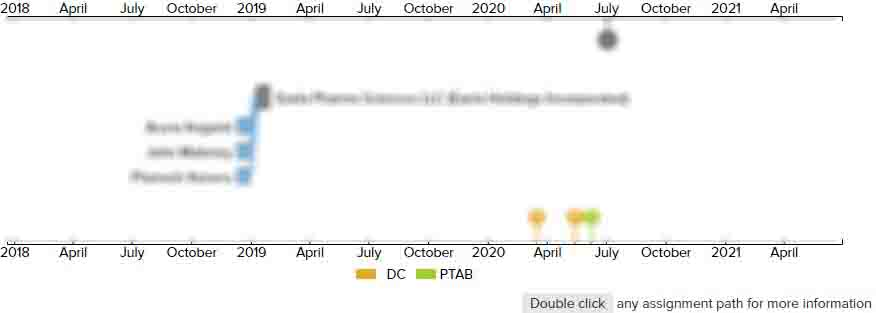Stereo decoding system

• US 9,374,117 B2
• Filed: 07/24/2012
• Issued: 06/21/2016
• Est. Priority Date: 07/25/2011
• Status: Active Grant
First Claim
Patent Images

1. A method for decoding a stereo multiplex signal, comprising:

• converting the stereo multiplex signal into a sum signal (L+R) and a difference signal (L−

R), where the sum signal and the difference signal are derived from a left stereo signal (L) and right stereo signal (R);

determining a first transfer function HL(f) and a second transfer function HR(f) from the sum signal (L+R) and the difference signal (L−

R), wherein the first transfer function HL(f) and the second transfer function HR(f) are each variable and adjustable;

adaptively filtering the sum signal (L+R) by modifying first filter coefficients according to the first transfer function to provide the left stereo signal (L); and

adaptively filtering the sum signal (L+R) by modifying second filter coefficients according to the second transfer function to provide the right stereo signal (R).

• 4 Assignments
Timeline View
Assignment View×
×